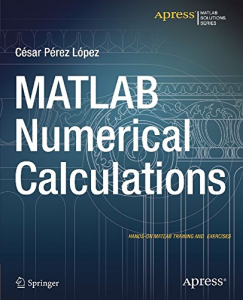# MATLAB Numerical Calculations (English Edition) 1st ed. Edition, Format Kindle

PLDZ-21 In Stock
\$ 5.00 USD
Description

MATLAB is a high-level language and environment for numerical computation, visualization, and programming. Using MATLAB, you can analyze data, develop algorithms, and create models and applications. The language, tools, and built-in math functions enable you to explore multiple approaches and reach a solution faster than with spreadsheets or traditional programming languages, such as C/C++ or Java. This book is designed for use as a scientific/business calculator so that you can get numerical solutions to problems involving a wide array of mathematics using MATLAB. Just look up the function you want in the book and you are ready to use it in MATLAB or use the book to learn about the enormous range of options that MATLAB offers.

MATLAB Numerical Calculations focuses on MATLAB capabilities to give you numerical solutions to problems you are likely to encounter in your professional or scholastic life. It introduces you to the MATLAB language with practical hands-on instructions and results, allowing you to quickly achieve your goals. Starting with a look at basic MATLAB functionality with integers, rational numbers and real and complex numbers, and MATLAB's relationship with Maple, you will learn how to solve equations in MATLAB, and how to simplify the results. You will see how MATLAB incorporates vector, matrix and character variables, and functions thereof. MATLAB is a powerful tool used to defined, manipulate and simplify complex algebraic expressions. With MATLAB you can also work with ease in matrix algebra, making use of commands which allow you to find eigenvalues, eigenvectors, determinants, norms and various matrix decompositions, among many other features. Lastly, you will see how you can write scripts and use MATLAB to explore numerical analysis, finding approximations of integrals, derivatives and numerical solutions of differential equations.

Recent Reviews Write a Review
0 0 0 0 reviews